# Formula for this curve?

• A
• TULC
In summary, the conversation involves finding a formula for an action potential, using it to graph in the Desmos graphics calculator, and exploring different approximations. Various functions are suggested, including a difference of two exponentials, a Crystal Ball function, and a piecewise continuous function. The conversation then shifts to finding a more precise solution, with a formula of 6*exp(-x^2*36)-exp(-(x-0.4)^2) being chosen and modified to fit the desired shape and values. The process involves adjusting parameters and subtracting 70 to get the baseline and peak heights right.

#### TULC

TL;DR Summary
Can someone help me figure out a formula for an action potential of a neuron? (attached)
I want to find a formula for an action potential (illustrated with the curve in the attachment). I would like to use the formula to graph this in Desmos graphics calculator. I don't have much of a math background, but a sine function comes to mind...I would like to get the precise shape though. See the source of the image here (top figure).

#### Attachments

•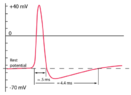Action Potential with values.png
5.2 KB · Views: 181
It doesn't look like any standard, closed-form, equation to me. What accuracy are you looking for? There are interpolation methods that can give a reasonable approximation, but they are messier than you might want.

•benorin and mfb
The difference of two exponentials can look roughly like that. Example: 6*exp(-x^2*36)-exp(-(x-0.4)^2)
To model the tail better a Crystal Ball function can be used.
sin(x)/x could be interesting, too.

But generally you won't get a really good approximations just with random functions unless you add an absurd amount of parameters.

•WWGD, benorin, dextercioby and 1 other person
Just eyeballing it, it might be possible to approximate it with the sum of a normal distribution and a piecewise continuous function like
\begin{equation*}
g(x)=\begin{cases}
0 \quad &\text{if} \, x \lt a_1 \\
\end{cases}
\end{equation*}
Where the parameters ##a_1##, ##a_2##, ##a_3##, and ##a_4## are TBD.

•benorin and sysprog
FactChecker said:
It doesn't look like any standard, closed-form, equation to me. What accuracy are you looking for? There are interpolation methods that can give a reasonable approximation, but they are messier than you might want.
Thank you. I would like to be able to at least convey the shape of the function to someone who is visually impaired (Desmos can sonify the functions). However, the higher the precision, the better. The values on the y-axis are of special interest. It would be good to capture the change in voltage from -70 mv to +40 mv (vertical)...

Thank you all...I will check these out.

FactChecker said:
Just eyeballing it, it might be possible to approximate it with the sum of a normal distribution and a piecewise continuous function like
\begin{equation*}
g(x)=\begin{cases}
0 \quad &\text{if} \, x \lt a_1 \\
\end{cases}
\end{equation*}
Where the parameters ##a_1##, ##a_2##, ##a_3##, and ##a_4## are TBD.
Interesting...I'll explore this.

Desmos is not really the ideal tool for this (and I have no idea if anything more suitable is available on the web) but I think your best chance may be modelling each part of the curve separately something like this:
https://www.desmos.com/calculator/v9hyi8qj0o

This looks similar to a 2nd order band-pass filter step response, as shown below. The parameter Q will adjust how oscillatory the curve is. The parameter ωo is just a scaling factor for time.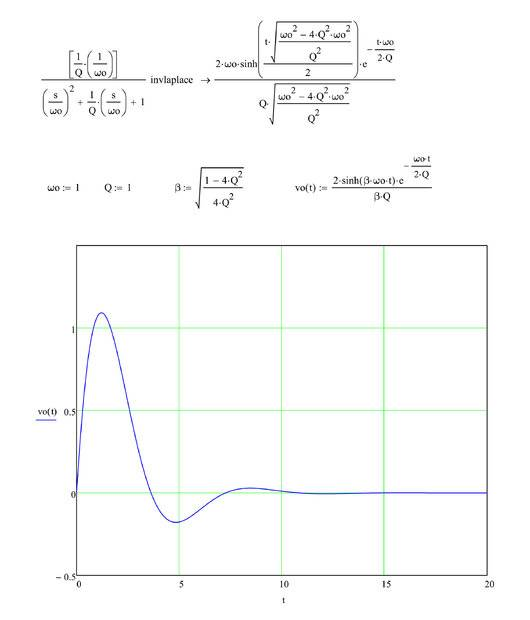•Delta2
The longer negative tail in your picture is a more nonlinear response. Probably best done in some piecewise approach. For example, the previous function where ωo is larger when the signal goes negative.

All of the above are very interesting, but it turns out I will need a more precise solution. I have been trying out different variations of mfp's formula 6*exp(-x^2*36)-exp(-(x-0.4)^2). I chose that one because it is easy to use in Excel. (I realize that some of you came up with more precise ones, but they were more difficult to use). So I was trying to modify it, but after many tries I was still unable to get a largely symmetrical curve with a *flat left tail and *an undershoot following the fall of the curve followed by a small rise to the original baseline level. The precise shape is important - if my description above is not clear enough, you can check the shape here. It would be good to to get key values right. That includes - 70 mv for the baseline (i.e., resting potential), +40 mV for the peak (action potential), -80 mV for the undershoot (hyperpolarization), then followed by return to baseline (around - 70 mV). I will continue working on this, though as I mentioned I don't have much of a math background. So this may take a while :) I would be so grateful if someone could kindly help me modify the above formula to get the precise shape and the values: -70 mv, + 40 mv, -80 mV followed by -70 mv. Many thanks!

You can't expect the shortest formulas to give the best approximations. The more complicated the function the better it can be tuned to your specific shape, generally.

Adding a baseline and making the amplitudes free parameters:
a + b*exp(-x^2*36) + c*exp(-(x-0.4)^2).

Far away from the peaks the exponentials evaluate to zero, so you can subtract 70 to get the baseline right: a=-70
The two exponentials have the maximum very close to 0 and the minimum close to 0.4.
At x=0.4 the first exponential term is exp(-5.76), i.e. it's negligible. The second one is just 1. To get the negative peak height right we need a+c = -80, i.e. c=-10.
At x=0 the first exponential term is just 1 and the second one is exp(-0.16). To get the peak height right we need a+b+exp(-0.16)c = 40 which leads to b = 118.

New plot

There is one additional degree of freedom: The offset of the second exponential - or alternatively the width of the first one. That determines how far the second exponential leaks into the first one.

Example with larger separation

-70 and -10 can stay the same, the 113 is the result of the updated calculation following the approach above.

•benorin and TULC
Thank you mfb! How should I acknowledge you if I create a working sonification based on the formula you proposed? I would be sharing it in an educational setting.

Not at all, or in any way you like, I don't mind.

•dlgoff
The action potential satisfy a 2nd order ODE according to the Hodgkin-Huxley model. See equation (30) of their seminal paper . This equation has no closed form solution, but can be solved numerically.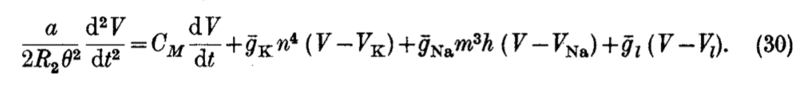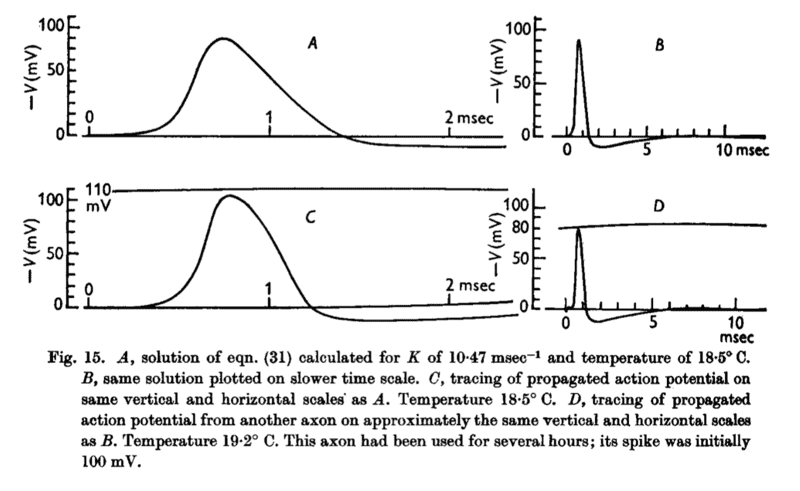. HODGKIN AL, HUXLEY AF. A quantitative description of membrane current and its application to conduction and excitation in nerve. J Physiol. 1952;117(4):500-544. doi:10.1113/jphysiol.1952.sp004764

•Delta2, WWGD, TULC and 1 other person
••WWGD, TULC, benorin and 1 other person
Am I correct in thinking that what you want is an audible tone with a frequency and/or volume that matches the curve? As we now have a differential equation we can use this to generate the curve, but not in Desmos.

It would be relatively simple to create a web page with controls to vary the parameters for the Hodgkin-Huxley model, numerically integrate the equation and generate corresponding audio (as well as graphical) output. If you are in an educational setting this would make an excellent project for a (bright) computer science high school student (although they might need help with the numerical integration).

I might have a look myself but my schedule is full for the next 10 days.

•TULC
mfb said:
...add an absurd amount of parameters.
Please can you give an example of this?

Approximating it with a polynomial of degree 100, for example. It will look great within the range you approximate, but (a) you have 101 parameters and (b) it will fail miserably outside the approximated range.

•benorin
mfb said:
..generally you won't get a really good approximations just with random functions unless you add an absurd amount of parameters.
To build a formula for a curve - no matter how intricate the curve is - I can always create a formula by adding parameters?

Thanks.

pairofstrings said:
To build a formula for a curve - no matter how intricate the curve is - I can always create a formula by adding parameters?
What you're really asking about is how to fit a polynomial or other curve to a set of data points. If there are a lot of data points or the curve is very complicated, what people do is to fit different polynomials to different portions of the curve. One technique uses what are called cubic splines.

Here's a wiki article you should look at -- https://en.wikipedia.org/wiki/Curve_fitting

If you have just two points, it's easy to find the equation of the line that contains those points. If you have three points, you can fit them to a quadratic function; i.e., a function of the form ##f(x) = ax^2 + bx + c##. Here the parameters are a, b, and c.

If you have four points, you can fit them to a cubic function; i.e., ##g(x) = ax^3 + bx^2 + cx + d##. If you have N points, a polynomial of degree N + 1 can be found.

Example for you: See if you can find the parameters a, b, and c so that the points (0, -1), (1.5, 1.25), and (2, 3) lie on the parabola ##y = ax^2 + bx + c##.

pairofstrings said:
To build a formula for a curve - no matter how intricate the curve is - I can always create a formula by adding parameters?

Thanks.
See the post just above yours.

A Fourier transform is another popular option.

For "reasonable" functions (a mathematician can tell you exact requirements, being at least piecewise continuous is a good start) you can always find arbitrary good approximations.

mfb said:
For "reasonable" functions (a mathematician can tell you exact requirements, being at least piecewise continuous is a good start) you can always find arbitrary good approximations.
So, if I approximate a curve by using the methods, it will suffice?
We don't need precise curve for the data points?

Suffice for what?
For a good approximation you only need a good approximation.

•benorin
Why do we approximate? Why shouldn't we be precise?
Are we trying to be precise by using the methods?
Band-pass filter comes to my mind.
The filter removes noise.
The other curve is being approximated?

#### Attachments

Last edited:
pbuk said:
Desmos is not really the ideal tool for this (and I have no idea if anything more suitable is available on the web) but I think your best chance may be modelling each part of the curve separately something like this:
https://www.desmos.com/calculator/v9hyi8qj0o
suggestion: TI-83 (emulators are available)

•benorin
pairofstrings said:
Why do we approximate?
Analytic solutions for real-life problems are rare. You almost always work with some approximation.

•benorin and sysprog
Deleted - see next post

Last edited:
TULC said:
Summary:: Can someone help me figure out a formula for an action potential of a neuron? (attached)

I want to find a formula for an action potential (illustrated with the curve in the attachment). I would like to use the formula to graph this in Desmos graphics calculator. I don't have much of a math background, but a sine function comes to mind...I would like to get the precise shape though. See the source of the image here (top figure).
Just to recap on this thread:
1. We want to create a frequency modulated audio output following a given curve for presentation to learners with a visual impairment.
2. The given curve is generated by a system of (4) differential equations (ODEs) without an analytical solution.
3. The Desmos website is capable of providing audio output, but it cannot solve differential equations.
One way of overcoming this is to find a (probably piecewise) approximation to the curve and input this into Desmos.

I think a better way is to create an application that can numerically solve ODEs, plot the results and produce an audio output. I have put something together to demonstrate this and it is available at https://avplot.com/#/hodgkin-huxley: an image of the graphical output is shown below. If this is useful, let me know and I will make it a bit smarter and add more functionality as time allows (e.g. the ability to enter arbitrary equations similar to Desmos, change the audio parameters etc). If it is not then it will probably die when the domain renewal comes up, although the (open) source will be available at https://github.com/avplot for as long as GitHub works.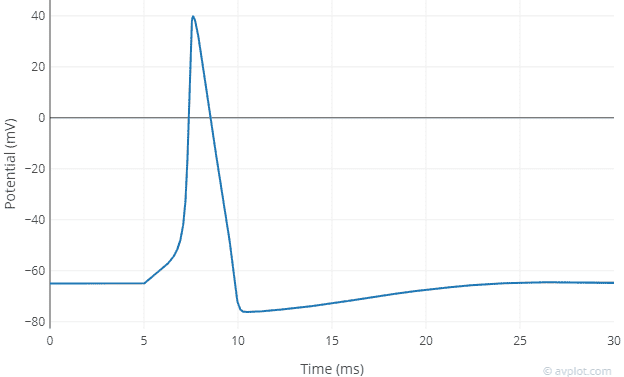•TULC and sysprog
Amazing input, thank you! I love the idea of using the Hodgkin-Huxley model for generating the visualization in an application that can also generate non-text audio. @pbuk It has been a while, would it be possible to add more functionalities to the application you created?

William Crawford said:
The action potential satisfy a 2nd order ODE according to the Hodgkin-Huxley model. See equation (30) of their seminal paper . This equation has no closed form solution, but can be solved numerically.
View attachment 287111

View attachment 287110

. HODGKIN AL, HUXLEY AF. A quantitative description of membrane current and its application to conduction and excitation in nerve. J Physiol. 1952;117(4):500-544. doi:10.1113/jphysiol.1952.sp004764
Thank you, William. That's very useful.

Thank you p
pbuk said:
Just to recap on this thread:
1. We want to create a frequency modulated audio output following a given curve for presentation to learners with a visual impairment.
2. The given curve is generated by a system of (4) differential equations (ODEs) without an analytical solution.
3. The Desmos website is capable of providing audio output, but it cannot solve differential equations.
One way of overcoming this is to find a (probably piecewise) approximation to the curve and input this into Desmos.

I think a better way is to create an application that can numerically solve ODEs, plot the results and produce an audio output. I have put something together to demonstrate this and it is available at https://avplot.com/#/hodgkin-huxley: an image of the graphical output is shown below. If this is useful, let me know and I will make it a bit smarter and add more functionality as time allows (e.g. the ability to enter arbitrary equations similar to Desmos, change the audio parameters etc). If it is not then it will probably die when the domain renewal comes up, although the (open) source will be available at https://github.com/avplot for as long as GitHub works.

View attachment 288064
This is excellent, thank you pbuk! Is it still possible to add the functionalities you mention in your post?

TULC said:
This is excellent, thank you pbuk! Is it still possible to add the functionalities you mention in your post?
Yes in theory: in practice I have very little free time at the moment. What would be the most desireable feature?

pairofstrings said:
To build a formula for a curve - no matter how intricate the curve is - I can always create a formula by adding parameters?